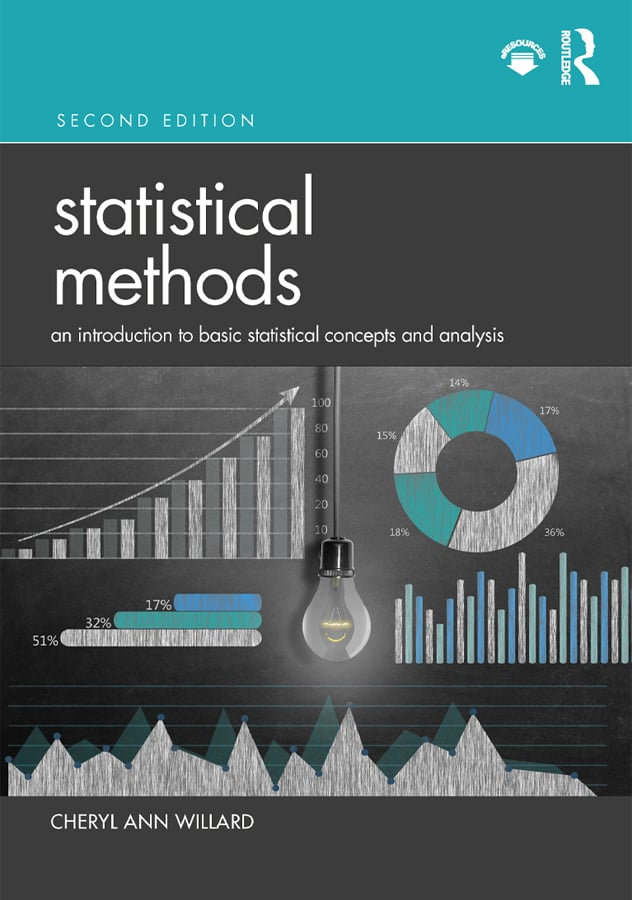# Statistical Methods

## An Introduction to Basic Statistical Concepts and Analysis, 2nd Edition

Routledge

304 pages | 137 B/W Illus.

Paperback: 9780367203528
pub: 2020-03-24
Available for pre-order. Item will ship after 24th March 2020
\$66.95
x
Hardback: 9780367203511
pub: 2020-03-24
Available for pre-order. Item will ship after 24th March 2020
\$200.00
x

FREE Standard Shipping!

### Description

Statistical Methods: An Introduction to Basic Statistical Concepts and Analysis, Second Edition is a textbook designed for students with no prior training in statistics. It provides a solid background of the core statistical concepts taught in most introductory statistics textbooks. Mathematical proofs are deemphasized in favor of careful explanations of statistical constructs.

The text begins with coverage of descriptive statistics such as measures of central tendency and variability, then moves on to inferential statistics. Transitional chapters on z-scores, probability, and sampling distributions pave the way to understanding the logic of hypothesis testing and the inferential tests that follow. Hypothesis testing is taught through a four-step process. These same four steps are used throughout the text for the other statistical tests presented including t tests, one- and two-way ANOVAs, chi-square, and correlation. A chapter on nonparametric tests is also provided as an alternative when the requirements cannot be met for parametric tests.

Because the same logical framework and sequential steps are used throughout the text, a consistency is provided that allows students to gradually master the concepts. Their learning is enhanced further with the inclusion of "thought questions" and practice problems integrated throughout the chapters.

New to the second edition:

• Chapters on factorial analysis of variance and non-parametric techniques for all data
• Additional and updated chapter exercises for students to test and demonstrate their learning
• Full instructor resources: test bank questions, Powerpoint slides, and an Instructor Manual

List of Tables

Preface

Acknowledgments

Introduction

Chapter 1. Introduction to Statistics

Chapter 2. Organizing Data Using Tables and Graphs

Chapter 3. Measures of Central Tendency

Chapter 4. Measures of Variability

Chapter 5. z-Scores and Other Standard Scores

Chapter 6. Probability and the Normal Distribution

Chapter 7. Sampling Distribution of Means

Chapter 8. Hypothesis Testing

Chapter 9. One-Sample t Test

Chapter 10. Two-Sample t Test: Independent Samples Design

Chapter 11. Two-Sample t Test: Related Samples Design

Chapter 12. Confidence Interval versus Point Estimation

Chapter 13. One-Way Analysis of Variance

Chapter 14. Factorial Analysis of Variance

Chapter 15. Correlation and Regression

Chapter 16. Chi-Square

Chapter 17. Nonparametric Statistics for Ordinal Data

Appendix A. Glossary of Statistical Terms

Appendix B. Glossary of Statistical Formulas

Appendix C. Answers to Odd Numbered End of Chapter Problems

Tables